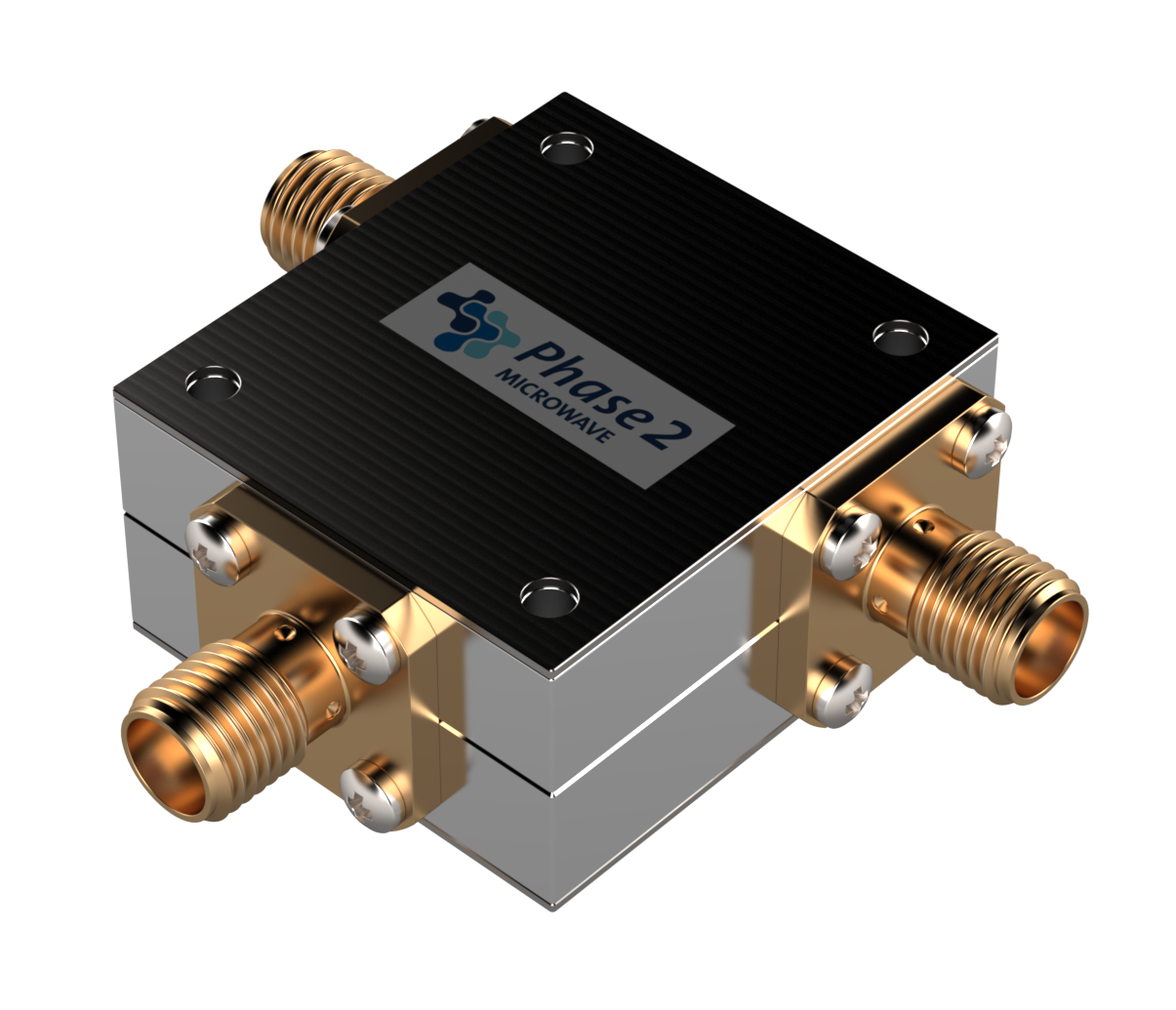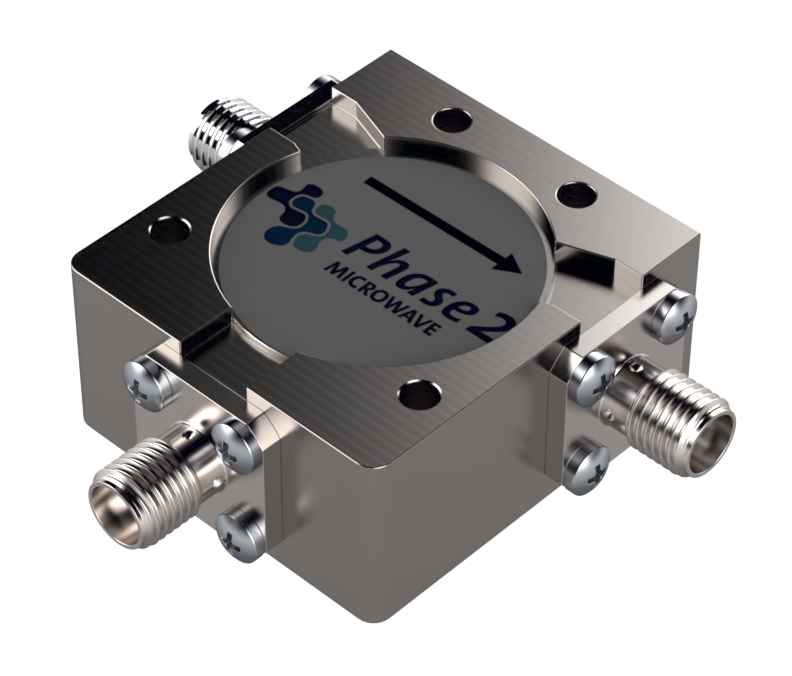Below is Phase2 Microwave's range of medium power
Circulators and Isolators, if you do not find what  you
 Circulators 3-6GHz Model FrequencyRange(GHz) Power(W) Insertion Loss (dB) Isolation(dB) VSWR Size(mm) Connector Data Sheet 3D Model Download006472 2.70-6.20 100 <=0.8 >=16 <=1.25 25.4x28.0x14.0 SMA Female006473 3.00-6.00 100 <=0.6 >=18 <=1.35 25.4x28.0x14.0 SMA FemaleCirculators 700MHz-3GHz Model FrequencyRange(GHz) Power(W) Insertion Loss (dB) Isolation(dB) VSWR Size(mm) Connector Data Sheet 3D Model Download006482 0.70-1.30 100 <=0.6 >=18. <=1.30 30.0x33.0x15.0 SMA Female006480 0.70-0.90 100 <=0.4 >=20 <=1.25 30.0x33.0x15.0 SMA Female006483 0.80-1.00 100 <=0.4 >=20 <=1.25 30.0x33.0x15.0 SMA Female006481 0.89-0.96 100 <=0.3 >=23 <=1.20 30.0x33.0x15.0 SMA Female006474 0.95-1.45 100 <=0.6 >=15 <=1.45 30.0x33.0x15.0 SMA Female006476 0.96-1.215 100 <=0.3 >=21 <=1.2 30.0x33.0x15.0 SMA Female006475 0.96-1.26 100 <=0.45 >=20 <=1.25 30.0x33.0x15.0 SMA Female006477 1.03-1.09 100 <=0.25 >=25 <=1.15 30.0x33.0x15.0 SMA Female006478 1.29-1.31 100 <=0.25 >=25 <=1.15 30.0x33.0x15.0 SMA Female006479 1.35-1.85 100 <=0.5 >=20 <=1.25 30.0x33.0x15.0 SMA Female006484 1.40-1.70 100 <=0.45 >=20 <=1.25 30.0x33.0x15.0 SMA Female006485 1.5-3.0 100 <=0.6 >=17 <=1.35 30.0x33.0x15.0 SMA Female006486 1.70-2.30 100 <=0.5 >=18 <=1.30 30.0x33.0x15.0 SMA Female006487 1.90-2.20 100 <=0.4 >=20 <=1.25 30.0x33.0x15.0 SMA Female006488 2.10-2.70 100 <=0.5 >=18 <=1.30 30.0x33.0x15.0 SMA Female006489 2.20-2.40 100 <=0.3 >=23 <=1.2 30.0x33.0x15.0 SMA Female006490 2.30-2.70 100 <=0.4 >=20 <=1.25 30.0x33.0x15.0 SMA Female006491 2.50-2.70 100 <=0.3 >=23 <=1.2 30.0x33.0x15.0 SMA Female006492 2.70-2.90 100 <=0.3 >=23 <=1.2 30.0x33.0x15.0 SMA Female006493 2.80-3.00 100 <=0.3 >=23 <=1.2 30.0x33.0x15.0 SMA Female